• 根据时域圆周移位特性 可得 即X1(k)={7,2+3j,-1-2j,-j} 5.2. 6 X(k)={7,2+3j,-1-2j,-j} 5.2.3 ... 8 中[x1(n) x2(n)]表示序列x1(n)和x2(n)的时域圆周卷积也称为循环卷积简称圆卷积其定义如下 5.2. 9 x1(n) 我们称
• 反式脂肪酸和卡诺拉油为标准，配制各种不同含量反式脂肪酸的标准溶液进行分析。通过傅里叶变换红外光谱仪和水平衰减全反射附件LabVIEW
• 以下为原创内容转载请注明地址 Fw=fourier(y):连续傅里叶变换函数 ft=ifourier(Fw,t):连续傅里叶变换函数 傅里叶变换举例如下： ...%Fw即为傅里叶变换后的子 ezplot(Fw);%可以画图检查一下 ...
以下为原创内容转载请注明地址
Fw=fourier(y):连续傅里叶变换函数
ft=ifourier(Fw,t):连续傅里叶逆变换函数
傅里叶变换举例如下：
syms t;
figure;
y = (sin(2pi(t-1)))/(pi*(t-1));
ezplot(y);%创建了函数y并画图现实
Fw=fourier(y);%Fw即为傅里叶变换后的式子
ezplot(Fw);%可以画图检查一下
傅里叶反变换举例如下：
syms t
Fw=sym(‘1/(1+jw)’)
ft=ifourier(Fw,t)%t为逆变换后的自变量
ezplot(ft);%可以画图检查一下
如果本文对你有帮助的话别忘了收藏点赞哦！


展开全文matlab
• 快速傅里叶变换-正文  计算... 　当用数字计算机计算信号序列x(n)的离散傅里叶变换时，它的正变换 　(1) 反变换(IDFT)是 　(2) 中、x(n)和X(k)可以是实数或复数。由上可见,要计
快速傅里叶变换-正文
计算离散傅里叶变换的一种快速算法,简称FFT。快速傅里叶变换是1965年由J.W.库利和T.W.图基提出的。采用这种算法能使计算机计算离散傅里叶变换所需要的乘法次数大为减少,特别是被变换的抽样点数N越多,FFT算法计算量的节省就越显著。 　　当用数字计算机计算信号序列x(n)的离散傅里叶变换时，它的正变换(1)
反变换(IDFT)是(2)
式中、x(n)和X(k)可以是实数或复数。由上式可见,要计算一个抽样序列就需要做N次复数乘法运算及N-1次复数加法运算。 　　计算离散傅里叶变换的快速方法，有按时间抽取的FFT算法和按频率抽取的FFT算法。前者是将时域信号序列按偶奇分排,后者是将频域信号序列按偶奇分排。它们都借助于的两个特点:一是的周期性；另一是的对称性，这里符号*代表其共轭。这样,便可以把离散傅里叶变换的计算分成若干步进行，计算效率大为提高。 　　时间抽取算法 　令信号序列的长度为N＝2M,其中M是正整数，可以将时域信号序列x(n)分解成两部分，一是偶数部分x（2n），另一是奇数部分x（2n+1），其中。于是信号序列x(n)的离散傅里叶变换可以用两个 N/2抽样点的离散傅里叶变换来表示和计算。考虑到和离散傅里叶变换的周期性,式(1)可以写成(3)
其中(4a)(4b)
由此可见，式(4)是两个只含有N/2个点的离散傅里叶变换，G(k)仅包括原信号序列中的偶数点序列，H(k)则仅包括它的奇数点序列。虽然k=0，1，2，…，N-1，但是G(k)和H(k)的周期都是N/2,它们的数值以N/2周期重复。 　　因为于是由式(3)和式(4)得到(5a)(5b)
因此,一个抽样点数为N 的信号序列 x(n)的离散傅里叶变换，可以由两个 N/2抽样点序列的离散傅里叶变换求出。依此类推，这种按时间抽取算法是将输入信号序列分成越来越小的子序列进行离散傅里叶变换计算，最后合成为N点的离散傅里叶变换。快速傅里叶变换

通常用图1中蝶形算法的信号流图来表示式(5)的离散傅里叶变换运算。例如，N＝8＝23的抽样点的信号序列x(n)的离散傅里叶变换,可用如图2所示的FET算法的信号流图来计算。由图可知 　　①　N=2M点的离散傅里叶变换的计算全由蝶形运算组成，需要M级运算,每级包括N/2个蝶形运算，总共有个蝶形运算。所以，总的计算量为次复数乘法运算和N log2N次复数加法运算。快速傅里叶变换

②　FFT算法按级迭代进行，计算公式可以写成(6)
N抽样点的输入信号具有N个原始数据x0(n),经第一级运算后，得出新的N个数据x1(n)，再经过第二级迭代运算，又得到另外N个数据x2(n)，依此类推，直至最后的结果x(k)＝xM(k)＝X(k)在逐级迭代计算中，每个蝶形运算的输出数据存放在原来存贮输入数据的单元中，实行所谓“即位计算”，这样可以节省大量存放中间数据的寄存器。 　　③　蝶形运算中加权系数随迭代级数成倍增加。由图2可以看出系数的变化规律。对于N=8,M=3情况,需进行三级迭代运算。在第一级迭代中，只用到一种加权系数;蝶形运算的跨度间隔等于1。在第二级迭代中，用到两种加权系数即、；蝶形运算的跨度间隔等于2。在第三级迭代中，用到4种不同的加权系数即、、、；蝶形运算的跨度间隔等于4。可见,每级迭代的不同加权系数的数目比前一级迭代增加一倍；跨度间隔也增大一倍。 　　④　输入数据序列x(n)需重新排列为x(0)、x(4)、x(2)、x(6)、x(1)、x(5)、x(3)、x(7),这是按照二进制数的码位倒置所得到的反序数，例如N=8中数“1”的二进制数为“001”，将其码位倒转变为“100”，即为十进制数“4”。 　　频率抽取算法 　按频率抽取的 FFT算法是将频域信号序列X(k)分解为奇偶两部分，但算法仍是由时域信号序列开始逐级运算，同样是把 N点分成N/2点计算FFT，可以把直接计算离散傅里叶变换所需的N2次乘法缩减到次。 　　在N＝2的情况下,把N点输入序列x(n)分成前后两半(7)
时间序列x1(n)±x2(n)的长度为N/2, 于是N点的离散傅里叶变换可以写成(8a)(8b)
频率信号序列X(2l)是时间信号序列x1(n)+x2(n)的N/2点离散傅里叶变换,频率信号序列X(2l+1)是时间信号序列【x1(n)-x2(n)】的N/2点离散傅里叶变换,因此,N点离散傅里叶变换的计算，通过两次加（减）法和一次乘法，从原来序列获得两个子序列，所以，频率抽取算法也具有蝶形运算形式。以2为基数的FFT基本蝶形运算公式为(9)
其计算量完全和时间抽取算法一样，即只需次乘法运算和Nlog2N次加(减)法运算。图3 表示N=8=23点的离散傅里叶变换的信号流图。由图可见,它以三级迭代进行即位计算,输入数据是按自然次序存放，使用的系数也是按自然次序，而最后结果则以二进制反序存放。快速傅里叶变换

实际上，频率抽取算法与时间抽取算法的信号流图之间存在着转置关系，如将流图适当变形，可以得出多种几何形状。 　　除了基2的FFT算法之外，还有基4、基8等高基数的FFT算法以及任意数为基数的FFT算法。 　　参考书目 　何振亚著：《数字信号处理的理论与应用》下册，人民邮电出版社，北京，1983。 　E.O.布里汉著，柳群译：《快速傅里叶变换》，上海科学技术出版社，1979。（E. O. Brigham, The　Fast Fourier Transform,Prentice Hall,Englewood Cliffs,New Jersey,1974.）
展开全文fft 算法
• ## 快速傅里叶变换

千次阅读 2016-11-30 14:27:13
离散傅里叶反变换为 FFT算法的基本思想是利用 的对称性和周期性，即 =- 和 （r为任意整数），通过将长序列分解为短序列，再由短序列的DFT组合来实现整个长序列的DFT，从而减少DFT的复数乘法和复数加法次数，提高...
快速傅里叶变化（FFT）是离散傅里叶变化（DFT）的快速算法。一个长度为N的有限长序列的DFT为

式中的 为 。离散傅里叶反变换为

FFT算法的基本思想是利用 的对称性和周期性，即 =- 和 （r为任意整数），通过将长序列分解为短序列，再由短序列的DFT组合来实现整个长序列的DFT，从而减少DFT的复数乘法和复数加法次数，提高DFT的运算速度和效率。将N点的长序列分解为短序列可以有多种方式，常用的有基2时间抽取FFT算法，基2频率抽取FFT算法，基4抽取FFT算法和分裂基FFT算法。基2抽取算法简单有效，基4抽取算法和分裂基抽取算法与基2抽取算法原理类似，但基4算法和分裂基算法效率更高，运算量更少。本文主要介绍基2抽取FFT算法。
1、基2时间抽取FFT算法：
基2时间抽取FFT算法是将N点序列x[n]按序号奇偶分解得到两个 点子序列 和 ,通过计算这两个子序列的DFT 和 实现 的DFT 。算法要点为N点序列x[n]对时域下标按奇数和偶数分解，即
（1）
对频域X[k]按前一半和后一半分解，即 。频域的前一半可由下式求得

由DFT的周期性和 的对称性有

-=
故频域的后一半可由下式求得

将前一半和后一半组合起来，即得原来的长序列x[n]的DFT 。
可对 和 继续按式(1)分解下去，直到每个子序列的长度为1为止。任何一个N= 点的DFT，可由通过M次分解，得到长度为1的子序列。对于N不是2的整数次幂的情况，可在序列后面添0补齐到2的整数次幂。每级分解运算可用一个蝶形运算流程图来表示，如图

下图是对一个长度为8的序列进行基2时间抽取FFT运算的流程图

直接计算N点序列的DFT需要N2次复数乘法，需要N(N-1)次复数加法。而用基2时间抽取FFT算法，则乘法次数为 ，加法次数 ，运算次数大大减少。
2、基2频率抽取FFT算法
基2频率抽取FFT算法则是对频域下标按奇数和偶数分解，对时域下标按前一半和后一半分解。 的DFT 可表示为

将 分解成 和 两个长度为 的子序列，如下：
（2）
则 的DFT 可按频率下标分奇数和偶数表示为

可继续将 和 按式(2)分解下去，直到每个子序列长度为1。任何一个N= 点的DFT，可由通过M次分解，得到长度为1的子序列。对于N不是2的整数次幂的情况，可在序列后面添0补齐到2的整数次幂。每级分解运算也可用一个蝶形运算流程图来表示，如图

下图是对一个长度为8的序列进行基2频率抽取FFT运算的流程图：

3、编程实现
Matlab有一个内置的函数fft()可以实现快速傅里叶变换，由于它是built-in函数，所以无法查看源码来判断它是应用的那种分解方式来实现的快速傅里叶变换。下面将用Matlab语言来编写实现类似fft()的快速傅里叶变换函数myfft()。本函数采用的FFT算法为基2时间抽取FFT算法，函数定义源码如下：
%----------------------------------
%myfft(A,M)有两个形参，A为要进行快速傅里叶变换的序列，M为序列长度对2的对数，
%即如果序列A[n]的长度为8，则M=3
function [A] = myfft(A,M)
N=2^M;                   %序列的长度为N
LH=N/2;                  %序列长度的一半
N1=N-2;
J=LH
for I=1:1:N1             %将输入序列A[n]按运算流程图第一列的顺序排好
if I<J
temp=A(I+1);
A(I+1)=A(J+1);
A(J+1)=temp;
end
K=LH;
while J>=K
J=J-K;
K=K/2;
end
J=J+K;
end
for L=1:1:M              %L表示序列分解层次，此次分解有M-L个子序列
B=2^(L-1);
for S=0:B-1          %执行第S个蝶形运算，每个子序列共有B个蝶形运算
p=S*2^(M-L);      %旋转因子的上标，即此时旋转因子为
for k=S:2^L:N-1   %执行各个子序列中的第S个蝶形运算，有M-L个子序列
temp=A(k+1)+A(k+B+1)*exp(-i*2*pi*p/N);
A(k+B+1)=A(k+1)-A(k+B+1)*exp(-i*2*pi*p/N);
A(k+1)=temp;  %蝶形运算
end
end
end
end
python语法简洁清晰，具有强大和丰富的三方库，能把其他语言(如C/C++,Matlab，java等)制作的各种模块轻松联结在一起，开发效率高，很适合快速开发实验原型以验证算法正确性和有效性。下面是基2时间抽取FFT算法的一个python版本实现。
#-------------------------------------
import numpy as np
def  FFT(x):
x =np.asarray(x, dtype=float)
N =x.shape
ifN%2>0:                                                      #输入序列的长度必须为2的幂
raiseValueError("size of x must be a power of 2")
elif N<= 2:                                                   # 子序列长度小于等于2时，直接计算
x =np.asarray(x, dtype=float)
N = x.shape
n = np.arange(N)
k = n.reshape((N, 1))
M = np.exp(-2j * np.pi * k * n / N)
eturn np.dot(M, x)
else:
X_even= FFT(x[::2])                               #偶序列继续分解
X_odd= FFT(x[1::2])                                              #奇序列继续分解
factor= np.exp(-2j * np.pi * np.arange(N/2) / N)        #旋转因子
returnnp.concatenate([X_even + factor* X_odd,         #返回序列频域前半部分
X_even - factor*X_odd])     #返回序列频域后半部分
4、函数测试和实验
快速傅里叶变换可以得到信号的频谱。通过对一个给定的信号进行快速傅里叶变换，观察它的频谱，并和Matlab内置函数fft()变换得到的结果进行对比，来对myfft()的功能进行测试。测试可用如下脚本来实现：
%----------------------------
%测试脚本，分别用myfft()和fft()对一个信号进行快速傅里叶变换，该信号由一个
%50Hz和120Hz的正弦信号叠加，频谱会在这50Hz和120Hz出现峰值。
Fs = 1000;                    % 采样频率
T = 1/Fs;                     % 采样周期
L = 5000;                     % 信号长度
t = (0:L-1)*T;               % 时间序号数组
% x(t)=0.7sin(2 *50t)+sin(2 *150t),为50Hz和150Hz正弦信号的叠加
x = 0.7*sin(2*pi*50*t) + sin(2*pi*150*t);
y = x + 2*randn(size(t));     % 给信号加上噪声
%画出x(t)和y(t)的图像
subplot(2,1,1)
plot(Fs*t(1:50),x(1:50))
title('Sinusoids Signal')
xlabel('time (milliseconds)')
subplot(2,1,2)
plot(Fs*t(1:50),y(1:50))
title('Signal Corrupted with Zero-Mean Random Noise')
xlabel('time (milliseconds)')
%对加了噪声的y(t)进行快速傅里叶变换
NFFT = 2^nextpow2(L);       %延长信号长度为最小的2的幂
y=[y zeros(1,2^nextpow2(L)-L)]; %延长信号长度，后面用0补齐
Y = myfft(y,log2(NFFT))/L; % 用自己实现的快速傅里叶变化myfft处理信号得到Y（f）
Y2 = fft(y,NFFT)/L;        %用Matlab官方实现的fft处理信号得到Y2(f）
f = Fs/2*linspace(0,1,NFFT/2+1);%频域横坐标
%对信号y(t)进行快速傅里叶变换，并画出的频谱
figure
subplot(2,1,1)
plot(f,2*abs(Y(1:NFFT/2+1)))       %

title('myfft()处理的y(t)频谱')% y(t)')
xlabel('Frequency (Hz)')
ylabel('|Y(f)|')
subplot(2,1,2)
plot(f,2*abs(Y2(1:NFFT/2+1)))       %
title('fft()处理的y(t)频谱')
xlabel('Frequency (Hz)')
ylabel('|Y2(f)|')
%对原信号x(t)进行快速傅里叶变换，并画出频谱
x=[x zeros(1,2^nextpow2(L)-L)]; %延长信号长度，后面用0补齐
X = myfft(x,log2(NFFT))/L;     % 用自己实现的快速傅里叶变化myfft处理信号得到X（f）
X2 = fft(x,NFFT)/L;             %用Matlab官方实现的fft处理信号得到X2(f）
figure
subplot(2,1,1)
plot(f,2*abs(X(1:NFFT/2+1)))       %

title('myfft()处理的x(t)频谱')%
xlabel('Frequency (Hz)')
ylabel('|X(f)|')
subplot(2,1,2)
plot(f,2*abs(X2(1:NFFT/2+1)))       %

title('fft()处理的x(t)频谱')
xlabel('Frequency (Hz)')
ylabel('|X2(f)|')
运行脚本，得到x(t)和y(t)的频谱，如图。可见，myfft()和Matlab内置函数fft()的处理结果一致，没有区别。频谱图在50Hz和150Hz出现峰值，幅值分别为0.63和1.0左右。

Python版的测试脚本如下
a=np.array([1,0,1,0,1,0,1,0])           #定义序列a[n]=[1,0,1,0,1,0,1,0]
b=[1,1,1,1,0,0,0,0]                         #定义序列b[n]=[1,1,1,1,0,0,0,0]
A=FFT(b)                                       #对a[n]进行快速傅里叶变换
B=FFT(a)                                      #对b[n]进行快速傅里叶变换
printA,”\n”,B                                  #输出两个序列的傅里叶变换的结果A[M]和B[m]
python版的傅里叶变换结果和Matlab自带的fft()变换结果对比如下

Python版的运行结果

Matlab版的运行结果
注意python版本的运行结果中，有”e-16”的结果，这是因为计算机浮点数的运算误差导致的，实际上可认为是0。可见，python实现的快速傅里叶变换函数的结果和Matlab的fft()变换结果一致，python实现的快速傅里叶变换结果正确无误。
5、快速傅里叶变换的应用
快速傅里叶变换可以将有限长或者周期离散时间信号从时域表示转换到频域表示。快速傅里叶变换除了可以得到一个信号的频谱外，还可以基于得到的频谱对信号进行一些频域上的处理，并通过快速傅里叶反变换得到经过处理后的信号。比如计算离散时间信号的线性卷积，滤波，信号压缩，降噪，谱估计等。FFT除了用于离散时间信号处理，也可以用于逼近连续时间信号的频谱。
下面脚本展示了利用快速傅里叶变换和反变换进行快速卷积计算
subplot(3,1,1);
n=0:16;
x=0.8.^n;      %x[n]=0.8^n的离散信号
stem(n,x);
xlabel('n');ylabel('x[n]');
subplot(3,1,2);
n=0:15;
y=[ones(1,10) zeros(1,6)];
stem(n,y);
xlabel('n');ylabel('y[n]')
subplot(3,1,3);
L=26;n=0:L-1;
X=fft(x,L);
Y=fft(y,L);
Z=X.*Y;      %在频域两信号的频谱相乘
z=ifft(Z,L);  %快速傅里叶反变换得到两信号的卷积
stem(n,z);
xlabel('n');
ylabel('z[n]')
脚本运行结果如图

在工程领域，FFT可以用于语音处理，工程传感器信号处理，特征提取等。
二维FFT和IFFT可应用到图像处理领域，比如图像降噪，模糊化，清晰化，轮廓化，压缩，还原等。快速傅里叶变换及其反变换是图像处理领域的重要处理手段，是进行其他各项处理的基础，应用场合很广。下面脚本展示了图像的二维快速傅里叶变换及其反变换，及基于快速傅里叶变换得到的频谱图，相位图等一系列频域信息。
A=rgb2gray(C);
B=fftshift(fft2(A));% 进行傅立叶变换
E=fft2(A);
D=ifft2(E);
subplot(331)
imshow(C);
title('原始图像');
subplot(332)
imshow(A);
title('原始灰度图像');
subplot(339)
imshow(abs(B),[]);
title('原始频谱图');
subplot(334)
imshow(log(abs(B)),[]);
title('取对数后的频谱图');
subplot(335)
imshow(angle(B),[]);
title('相位图');
subplot(336)
imshow(real(B),[]);
title('实部图');
subplot(337)
imshow(imag(B),[]);
title('虚部图');
subplot(338)
imshow(abs(E),[]);
title('fft2频谱图');
subplot(333)
imshow(D,[]);
title('经快速傅里叶逆变换得到的灰度图');
下面为脚本运行的结果，可见经快速傅里叶反变换后，可以从图像的频域表示得到时域表示，即重新得到一张图像。由于此脚本没有对图像做其他处理，所以快速傅里叶反变换得到的图形与原来的灰度图像一致。

6、总结
快速傅里叶变换大大简化了运算量，并且算法具有迭代和分治的特点，特别适合计算机实现，使得用计算机对离散时间信号进行快速处理成为现实。配合采样定理和信号采样及重构等方法，使得快速傅里叶变换可以进一步推广到连续时间信号处理，大大提高了信号处理的效率和速度。在语音处理领域，傅里叶变换可以用于语音识别，音频分离，降噪等应用。在图像应用领域，快速傅立叶变换的物理意义是将图像的灰度分布函数变换为图像的频率分布函数，傅立叶逆变换是将图像的频率分布函数变换为灰度分布函数。而灰度分布函数即为灰度图的表达。快速傅里叶变换使得对图像可以用频域处理的方法来处理，对基于计算机的图像处理应用的可行性和实时性具有重大意义，是数字信号处理的基石。

展开全文• 有限长的离散变换：离散傅里叶变换 Finite-Length Discrete Transforms： DFT(Discrete Fourier Transform) ...那么对于有限长度的序列x[n]（0≤n≤N-1），其正交变换与正交反变换的系数为： 2.DFT的定义
有限长离散变换：离散傅里叶变换

Finite-Length Discrete Transforms： DFT(Discrete Fourier Transform)

1.正交变换（Orthogonal Transforms）

若对于基序列 ψ[k,n]，有：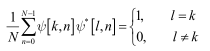那么对于有限长度的序列x[n]（0≤n≤N-1），其正交变换与正交反变换的系数为：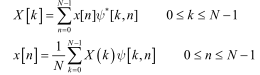2.DFT的定义式

若使用旋转引子(twiddle factor)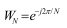来表示DFT，那么有：

正变换（DFT）：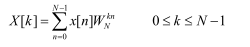反变换（IDFT）：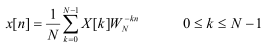3.DFT与DTFT的关系

离散傅里叶变换（DFT）X[k]是由在序列x[n]的DTFT的区间0≤ω≤2π以间隔2π/N等间隔取样得到的，即：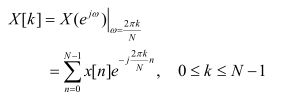展开全文• 定义 记连续时间信号的采样为，其傅里叶变换为 这就是采样序列的DTFT： 为方便起见，通常将采样间隔T归一化，则有 ...上即为的离散时间傅里叶变换。它的反变换为： 考虑到DTFT的周file c
• 1、理论 首先给出傅里叶变换的旋转、平移、比例放大特性，及如何利用这些特性进行图像配准。...通过对互能量谱进行反变换，就可得到一个冲击函数。此函数在偏僻位置处有明显的尖锐峰值。其他位...
• 点击上方“蓝字”关注我们第二章Z变换与离散时间傅里叶变换(DTFT)01序列的Z变换(1)Z变换，Z反变换的定义(2)Z变换的收敛域(3)四种典型序列的Z变换及其收敛域：有限长序列(如何判断收敛域含不含0和无穷大)、右边序列...
• 在图像处理中，傅里叶变换应该是二维的，而且，是离散的，即：二维DFT。一个图像尺寸为的函数的离散...给出,可以通过傅里叶反变换来计算得到f(x,y),即：  （2） 其中，x=0,1,...,M-1,y=0,1,...,N-1;上面两个子共同
• DFT的的正变换和反变换分别为（1）和（2）。假设有N个数据，则计算一个频率点需要N次复数乘法和N-1次复数加法，整个DFT需要N*N次复数乘法和N（N-1）次复数加法；由于一次的复数乘法需要进行4次的实数乘法和2次的复...
• 其中c12和c21分别是f1在f2上的分量系数和f2在f1上的分量系数（以上那个题目可能是印了） 再放一个方均误差的问题： 关于这个方均误差，它的定义是这样的： 不用我说，你拿这个子去求上面那道题目，不论是...
• 将上面两式写成矩阵形式，则有：对于正变换：对于反变换：在推导关系式时，经常需要用到的关系式：2 DFT与DTFT的关系通过采样从DTFT得到DFT已知DTFT的正变换为：对比DFT的变换式：可知：N点DFT 就是 对DTFT以采样...
• ## 傅丽叶变换（二）

千次阅读 2012-12-01 20:55:37
傅丽叶变换（二） ——(java)算法实现 离散傅里叶变换 离散傅里叶变换使得数学方法与计算机技术建立了联系，这就为傅里叶变换这样一个数学工具在实用中开辟了一条宽阔...傅里叶反变换定义由下来表示 由(1)
• 离散傅里叶变换 离散傅里叶变换使得数学方法与计算机技术建立了联系，这就为傅里叶变换这样一个数学工具在实用中开辟了...傅里叶反变换定义由下来表示 由(1)和(2)可见，离散傅里叶变换是直接处理离散时间信号的傅
• 研究过离散傅里叶变换的，都会觉得离散余弦变换的会更简单，不过不知道为什么网上却...二维DCT的反变换公式为： 以上各中的系数：   基于DCT的JPEG图像压缩编码理论算法 基于DCT编码的JPEG编码压
• 研究过离散傅里叶变换的，都会觉得离散余弦变换的会更简单，...二维DCT的反变换公式为： 以上各中的系数：   基于DCT的JPEG图像压缩编码理论算法 基于DCT编码的JPEG编码压缩过程框图，如图1所示。 ...
• 图像处理中常用的正交变换除了傅里叶变换外，还有其他一些有用的正交变换,其中离散余弦就是一种。离散余弦变换表示为DCT(DiscreteCosineTransformation)，常用于图像处理和图像识别等。一维离散余弦变换变换(1) (2...
• 研究过离散傅里叶变换的，都会觉得离散余弦变换的会更简单，不过不知道为什么网上却没有像离散傅里叶变换那样多的介绍，还得下次找...二维DCT的反变换公式为： 以上各中的系数：   基于DCT的JPEG图像压
• 傅里叶变换一样，拉普拉斯变换的性质也可以实现对更多信号拉普拉斯变换的求解，除此之外，之前提到的求解拉普拉斯反变换的分部分法以及留数法，也是在拉普拉斯变换具有线性和齐次性的前提下。这一部分内容将对...信号处理
• 几个快速傅立叶变换算法  2008-03-09 19:25:47| 分类： ...DFT的的正变换和反变换分别为（1）和（2）。假设有N个数据，则计算一个频率点需要N次复数乘法和N-1次复数加法，整个DFT需要N*N次复数乘法和
• 反变换： 一维离散的傅里叶变换： 反变换：变过去就一定要变回来 二维离散傅里叶变换： 反变换： 由于傅里叶变换结果一般都是复数，由此可以用极坐标表示： 傅里叶谱： 相角： 能量谱： ...
• 反变换可以定义为： 上述这两个子，就反映了通过一个函数可以做变换求到其傅里叶变换，或已知傅里叶变换可以完全地求出原始函数。 单变量离散函数f(x)（其中x=0,1,2,…,m-1）的傅里叶变换由以下等式给出： 同样...计算机视觉